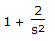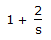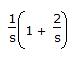# Chemical Engineering - Process Control and Instrumentation

### Exercise :: Process Control and Instrumentation - Section 3

21.

A process is initially at steady state with its output y=l for an input u=l. The input is suddenly changed to 2 at time t=0. The output response is y(t)=1+2t. The transfer function of the process is

 A. 2/s B.C.D.Explanation:

No answer description available for this question. Let us discuss.

22.

The term analogous to the electrical current in a thermal system is the

 A. temperature difference B. heat flow rate C. heat content in the system D. none of these

Explanation:

No answer description available for this question. Let us discuss.

23.

Reset rate is the another term used for __________ time.

 A. dead B. integral C. derivative D. none of these

Explanation:

No answer description available for this question. Let us discuss.

24.

Continuous measurement of moisture in paper is done by

 A. sling psychrometer. B. hair-hygrometer. C. weighing. D. high resistance Wheatstone's bridge circuit.

Explanation:

No answer description available for this question. Let us discuss.

25.

Psychrometer determines the

 A. humidity of gases B. moisture content of solids C. water of crystallisation D. hygroscopic nature of solids